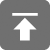# 工学1号馆

home

## C语言指针的长度和类型

Wu Yudong    June 27, 2015     C   468

#include<stdio.h>
#include<math.h>
#include<stdlib.h>
#include<stddef.h>
struct p{
int n;
float f;
};
int main()
{
struct p *sptr;
printf("sizeof *char: %d\n", sizeof(char*));
printf("sizeof *int: %d\n", sizeof(int*));
printf("sizeof *float: %d\n", sizeof(float*));
printf("sizeof *double: %d\n", sizeof(double*));
printf("sizeof *struct: %d\n", sizeof(sptr));
return 0;
}• size_t：用于安全地表示长度
• ptrdiff_t：用于处理指针算术运算
• intptr_t：用于存储指针地址
• uintptr_t：用于存储指针地址

### size_t类型

size_t 类型是标准C库中定义的，应为unsigned int，在64位系统中为 long unsigned int。 C语言中，此类型位于头文件stddef.h中。它是一个与机器相关的unsigned类型，其大小足以保证存储内存中对象的大小，它的目的是提供一种可移植的方法来声明与系统中可寻址的内存区域一致的长度：

size_t类型用作sizeof操作符的返回类型，同时也是很多函数的参数类型，包括malloc和strlen

### ptrdiff_t类型

ptrdiff_t是C99标准库中定义的一个与机器相关的数据类型，定义在stddef.h这个文件内。ptrdiff_t类型变量通常用来保存两个指针减法操作的结果。
ptrdiff_t通常被定义为long int类型，size_t 是unsigned 类型,而 ptrdiff_t 则是 signed 整型。

#include<stdio.h>
#include<stddef.h>
#include<string.h>
int main(void)
{
char str[] = "Hello world!";
char *pstart = str;
char *pend = str + strlen(str);
ptrdiff_t difp = pend - pstart;
printf("%d\n", difp);
return 0;
}

### intptr_t与uintptr_t类型

intptr_t与uintptr_t类型用来存放指针地址，它们提供了一种可移植且安全的方法声明指针，而且与系统中使用的指针的长度相同，对于把指针转化为整数形式很有用。uintptr_t是intptr_t的无符号版本

/* Types for void *' pointers.  */
#if __WORDSIZE == 64
# ifndef __intptr_t_defined
typedef long int               intptr_t;
#  define __intptr_t_defined
# endif
typedef unsigned long int    uintptr_t;
#else
# ifndef __intptr_t_defined
typedef int                    intptr_t;
#  define __intptr_t_defined
# endif
typedef unsigned int        uintptr_t;
#endif

#include <stdio.h>
#include <stdlib.h>
#include <unistd.h>
#include <stdint.h>
#include <string.h>
#include <assert.h>

#define ID_STR_LEN   12
#define NAME_STR_LEN 10

typedef struct student
{
char id[ID_STR_LEN];
char name[NAME_STR_LEN];
uint8_t age;
}student;

student * create_student()
{
student *stu = (student *)malloc(sizeof(student));
if (stu == NULL)
return NULL;
memset(stu, 0, sizeof(student));
return stu;
}

void *free_student(student *stu)
{
if (stu)
free(stu);
return 0;
}

static void init_student(student * stu)
{
assert(stu);
const char *id = "2013112210";
const char *name = "Anker";
uint8_t age = 21;
memcpy(stu->id, id, strlen(id));
memcpy(stu->name, name, strlen(name));
stu->age = age;
}

static int handle_student(intptr_t handle)
{
if (handle == 0)
{
return -1;
}
student *stu = (student*)handle;
printf("id: %s\n", stu->id);
printf("name: %s\n", stu->name);
printf("age: %u\n", stu->age);
return 0;
}

int main(void)
{
student *stu;
stu = create_student();
init_student(stu);
//将指针转换为intptr_t类型
intptr_t handle = (intptr_t)stu;
handle_student(handle);
free_student(stu);
return 0;
}`

http://www.cnblogs.com/Anker/p/3438480.html